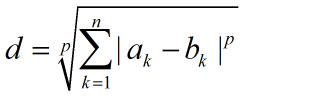## sklearn.neighbors.KNeighborsClassifier的k-近邻算法使用介绍 转

o
osc_wws45aot

``class sklearn.neighbors.KNeighborsClassifier(n_neighbors=5, weights=’uniform’, algorithm=’auto’, leaf_size=30, p=2, metric=’minkowski’,metric_params=None, n_jobs=None, **kwargs)``

## 1,KNeighborsClassifier参数介绍

### n_neighbors: int，默认值为5

表示k-nn算法中选取离测试数据最近的k个点，

### weight: str or callable，默认值为uniform

表示k近邻点都分类结果的影响，一般的情况下是选取k近邻点中类别数目最多的作为分类结果，这种情况下默认k个点的权重相等，但在很多情况下，

k近邻点权重并不相等，可能近的点权重大，对分类结果影响大。

默认值为uniform，还可以是distance和自定义函数

• 'uniform'：表示所有点的权重相等
• 'distance'：表示权重是距离的倒数，意味着k个点中距离近的点对分类结果的影响大于距离远的点
• [callable]：用户自定义函数，接受一个距离数组，返回一个同维度的权重数

### algorithm：{'ball_tree','kd_tree','brute','auto'}

计算找出k近邻点的算法

• 'ball_tree'：使用BallTree维数大于20时建议使用
• 'kd_tree'：使用KDTree，原理是数据结构的二叉树，以中值为划分，每个节点是一个超矩形，在维数小于20是效率高
• 'brute'：暴力算法，线性扫描
• 'auto'：自动选取最合适的算法

note：在稀疏的输入数据上拟合，将使用'brute'覆盖此参数

### leaf_size：int，默认值为30

用于构造BallTree和KDTree

leaf_size参数设置会影响树构造的树构造和询问的速度，同样也会影响树存储需要的内存，这个值的设定取决于问题本身

### p：int，默认值为2

• 1：使用曼哈顿距离进行度量
• 2：使用欧式距离进行度量

### metric : string or callable, 默认使用'minkowski'(闵可夫斯基距离)，度量函数其中p是一个变参数，也就是上一个介绍的参数p

当p=1时，就是曼哈顿距离

当p=2时，就是欧氏距离

当p→∞时，就是切比学夫距离

### metric_params : dict, 默认为None

度量函数的其他关键参数，一般不用设置

### n_jobs : int or None, 默认None

用于搜索k近邻点并行任务数量，-1表示任务数量设置为CPU的核心数，即CPU的所有core都并行工作，不会影响fit(拟合)函数

https://en.wikipedia.org/wiki/K-nearest_neighbor_algorithm

 `fit`(self, X, y) 使用X作为训练集作为便签集，来拟合模型 `get_params`(self[, deep]) 获得估计器的参数 `kneighbors`(self[, X, n_neighbors, …]) 查找X数组X数组中所有点的K邻居点 `kneighbors_graph`(self[, X, n_neighbors, mode]) 计算X中每个点的K邻居权重图 `predict`(self, X) 预测X数据集中每个点对应的标签 `predict_proba`(self, X) 返回X数据集中对应每个标签的概率估计值 `score`(self, X, y[, sample_weight]) 返回给定数据集和标签的平均准确率 set_params(**params) 设置估计器的参数

``__init__(self, n_neighbors=5, weights=’uniform’, algorithm=’auto’, leaf_size=30, p=2, metric=’minkowski’, metric_params=None, n_jobs=None, **kwargs)``

[source]

`fit`(selfXy)

X：{类似数组，稀疏矩阵，BallTree，KDTree}

训练集：如果是数组或者矩阵，形状为[n_samples, n_features]，如果参数metric='precomputed'，形状为[n_samples, n_samples]

y：{类似数组，稀疏矩阵}

标签集：形状为[n_samples]或者[n_samples, n_outputs]

`get_params`(selfdeep=True)

deep：boolean，可选

如果为True，返回估计器的参数，以及包含子估计器

Returns：mapping of string to any

返回Map变量，内容为[参数值:值, 参数值:值, ...]

kneighbors(X=None,n_neighbors=None,return_distance=True)

X：类型数组，形状为 (n_query, n_features)，如果参数metric == ‘precomputed’形状为(n_query, n_indexed)

在没有提供查询点的情况下，则返回有下标点的邻居们，这种情况下，没有考虑查询点的邻居们

n_neighbors：int

返回的邻居点的个数（默认使用改造器是设定的n_neighbors值

return_distance：boolean，可选。 默认为True

如果为False，距离值就不会返回

Returns：

dist：数组

当return_distance=True是，返回到每个近邻点的距离

ind：数组

返回近邻点的下标

例子

``````from sklearn.neighbors import NearestNeighbors
samples = [[0., 0., 0.], [0., .5, 0.], [1., 1., .5]]
neigh = NearestNeighbors(n_neighbors=1)
neigh.fit(samples)
print(neigh.get_params())
print(neigh.kneighbors([[1., 1., 1.]]))``````

{'algorithm': 'auto', 'leaf_size': 30, 'metric': 'minkowski', 'metric_params': None, 'n_jobs': None, 'n_neighbors': 1, 'p': 2, 'radius': 1.0}
(array([[0.5]]), array([], dtype=int64))

``````X = [[0., 1., 0.], [1., 0., 1.]]
print(neigh.kneighbors(X, return_distance=False))``````

[,]

[,]表示，第一个查询点的近邻点下标为1，同样第二个查询点的近邻点下标为2

`kneighbors`(selfX=Nonen_neighbors=Nonereturn_distance=True)

X：类似数组，形状为 (n_query, n_features)，如果参数metric == ‘precomputed’，形状为 (n_query, n_indexed)

一个或者多个查询点，如果没有提供，则返回每个有下标的邻居们

n_neighbors int

每个查询的邻居数量(默认使用拟合时设置的n_neighbors)
mode {‘connectivity’, ‘distance’}, 可选

返回矩阵类型：'connectivity'，返回0和1组成的矩阵，'distance'，返回点与点之间的欧几里得距离

A：CSR格式的稀疏矩阵，形状为[n_samples, n_samples_fit]

n_samples是拟合过程中样例的数量，A[i, j]是i到j的权重

关联：`kneighbors_graph`

``````from sklearn.neighbors import NearestNeighbors
X = [, , ]
neigh.fit(X)
print(A.toarray())``````

[[1. 0. 1.]
[0. 1. 0.]
[1. 0. 1.]]

`predict`(selfX)

X：类似数组，形状为(n_query, n_features)，如果参数metric == 'precomputed'，形状为(n_query, n_indexed)

待测试数据

y：形状为[n_samples]或者为[n_samples, n_outputs]

每个待测试样例的类别标签

`predict_proba`(selfX)

X：类似数组，形状为(n_query, n_features)，如果参数metric == 'precomputed'，形状为(n_query, n_indexed)

带测试样例

p：形状为[n_samples, n_classes]，或者n_outputs列表

输出每个样例对于每个类别的概率估计值，类别按照字典顺序排序

``````from sklearn.neighbors import KNeighborsClassifier
X = [, , , ]
y = [0, 0, 1, 1]
neigh = KNeighborsClassifier(n_neighbors=3)
neigh.fit(X, y)
print(neigh.predict([[1.1]]))
print(neigh.predict_proba([[1.1]]))``````


[[0.66666667 0.33333333]]

`score`(selfXysample_weight=None)

X：类似数组，形状为 (n_samples, n_features)

测试数据
y：类似数组，形状为(n_samples)或者(n_samples, n_outputs)

X对应的正确标签

sample_weight : 类似数组,形状为[n_samples], 可选

样例的权重

score float

self.predict(X)关于y的平均准确率

``````from sklearn.neighbors import KNeighborsClassifier
X = [, , , ]
y = [0, 0, 1, 1]
neigh = KNeighborsClassifier(n_neighbors=3)
neigh.fit(X, y)
print(neigh.predict([[1.1], [2.1], [3.1]]))
print(neigh.score([[1.1], [2.1], [3.1]], [0, 1, 0]))[0 1 1]0.6666666666666666``````

可以看出3个测试数据，预测类别有2个正确，1个错误，所有准确率为0.6666666666666666

`set_params`(self**params)

self

o

### osc_wws45aot

【学习笔记】分类算法-k近邻算法

k-近邻算法采用测量不同特征值之间的距离来进行分类。 优点：精度高、对异常值不敏感、无数据输入假定 缺点：计算复杂度高、空间复杂度高 使用数据范围：数值型和标称型 用例子来理解k-近邻算...

osc_127lqd1g
2019/03/17
2
0

osc_m8nx82a1
2019/06/29
2
0
sklearn.neighbors.KNeighborsClassifier(k近邻分类器)

KNeighborsClassifier参数说明(nneighbors=5, weights='uniform', algorithm='auto', leafsize=30, p=2, metric='minkowski', metricparams=None, njobs=None, **kwargs) n_neighbors：所选用......

2019/12/27
0
0
sklearn.neighbors.KNeighborsClassifier(k近邻分类器)

KNeighborsClassifier参数说明(nneighbors=5, weights='uniform', algorithm='auto', leafsize=30, p=2, metric='minkowski', metricparams=None, njobs=None, **kwargs) n_neighbors：所选用......

osc_lrhgywny
2019/12/27
1
0
web安全之机器学习入门——3.1 KNN/k近邻

osc_962c7vo6
2019/04/08
2
0

Linux就该这么学
37分钟前
20
0
C++ 从基本数据类型说起

osc_sxdofc9c
37分钟前
9
0

37分钟前
10
0
2020，最新Model的设计-APP重构之路

osc_mfzkzkxi
38分钟前
4
0

osc_gfpedeca
39分钟前
21
0